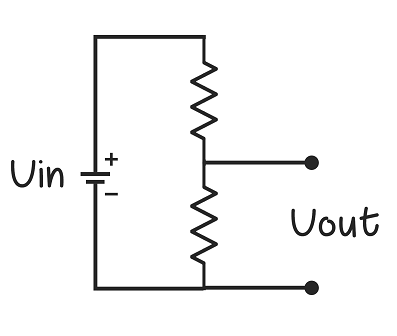## Introduction to Electronics

Electronics deals with the use of circuits that involve various electrical components. Everything from the clock you see to find time to the smartphone in your hand connecting you to your friends every second are the applications of it. In this section, we will give a brief overview of the electronics that we need to get started with working on the book.

If you are a beginner, this video series on YouTube is a great way to start- "Building Electronic Circuits"

It starts off by giving you an overview of the basics of the electronic components generally used and teaches you how to work on some cool projects such as infra red sensors and wearable circuits. Watching and practicing the circuits in the first six videos in this series would give you a decent level of understanding to continue reading the sections below. These videos are optional to watch for people who have worked on electronic circuits before.

Next, we explain a simple circuit that is used very commonly while trying to solve mobile games.

### Voltage Divider CircuitA voltage divider circuit is commonly used in many electronic circuits for a wide range of applications including adjusting level of signal, measurement of voltages, etc. It is used in multimeter and wheatstone bridge. It produces an output voltage which is a fraction of the input voltage. The value of the output voltage depends on the values of the resistors used.

The output voltage of the above circuit can be calculated by the following equation. Read about ohm's law and work on some examples to know where we get the equation from.

Vout = ((R2) / (R1 + R2)) * Vin

We will use this circuit in a following section on sensors.

Next, let us learn about how to use microcontrollers in building electronic circuits, specifically, Arduino.